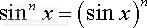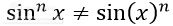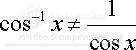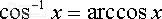sections

# Common Input Errors with Your Calculator that You Probably Didn’t Notice

You studied so hard only to be failed by your calculator – ouch!Engineers, whatever field you specialize in, have a universal best friend: a calculator. Almost all the time – when your fingers can no longer perform a trigonometric function – you depend on your life with the calculators in solving problems. But there will be cases that you will be faced with errors you didn’t know you have made. And that could cost you a failing grade or even your job. Maybe even lives of people.

While there are so many kinds of calculators, here are the common input errors that you may have encountered in any calculator. It’s best that you read your calculator manuals, but most likely you can find those input errors here:

## Using [–] instead of [(-)] to indicate that a number is negative

Common rookie error. You use the [–] to subtract, and [(-)] to denote a negative number. There is a difference. When you use [–] instead of [(-)] in the interior of an expression to denote a negative number, the calculator responds with the syntax error message.

## Indicating the order of operations incorrectly by using parentheses

In your calculator, –3^2 is not the same as (–3)^2. The calculator first performs the operation in parentheses, then it squares the number. If there is a negative outside the parentheses, it first performs the squaring, then the operation of a negating a number. That’s how crucial the order of operations is in evaluating expressions.Photo via Extreme Tech

## Entering an angle in degree in radian mode

In the calculator, trigonometric functions can be used with two modes: degree or radian. Just make sure that you are using the degree mode or radian mode, depending on what you’re required. Changing the mode depends on the settings of your calculator.

Read more  Why Do Students Choose Chemical Engineering

## Placing the powers for trigonometric functions

It can only besince. Remember the order of operations? Yeah, that’s it. All trigonometric functions hold this notational idiosyncrasy.

## Entering the inverse trigonometric notation

Here’s another: just because the trigonometric function is raised to a negative number, which you know is an inverse, it is not an exponent; in the calculator, the inverse of the cos(x) function is not 1/cos(x). In short,but.

Since you are already here, let’s also discuss the kinds of calculator errors that you usually encounter according to Casio.

### Ma ERROR or Math ERROR

Cause: The calculation result is outside of the allowable calculation range or you are trying to perform an illegal mathematical operation (such as division by zero).

Action: Check your input values and adjust them to allow the calculation result to be within the allowable range. If you are using memory for your calculation, make sure that you check the memory value.

### Syn ERROR or Syntax ERROR

Cause: The calculation expression is the wrong syntax, or the program you are using contains a syntax error.

Action: Press the <right> or <left> key to display the location of the error, and correct it.

### Stk ERROR or Stack ERROR

Cause: The calculation you are performing has caused the capacity of the numeric stack or the command stack to be exceeded.

Action: Simplify your calculation expression. You could also split your calculation expression into two or more parts and execute the parts separately.

### Mem ERROR

Cause: You executed a matrix, graphing, or some other operation that caused remaining memory capacity to be exceeded.

Read more  Do Elite Schools Matter in High Paying Jobs?

Action: Delete memory data you no longer need. Make sure that the number of variables used in your expression does not exceed the number of variables that are available.

You are welcome.